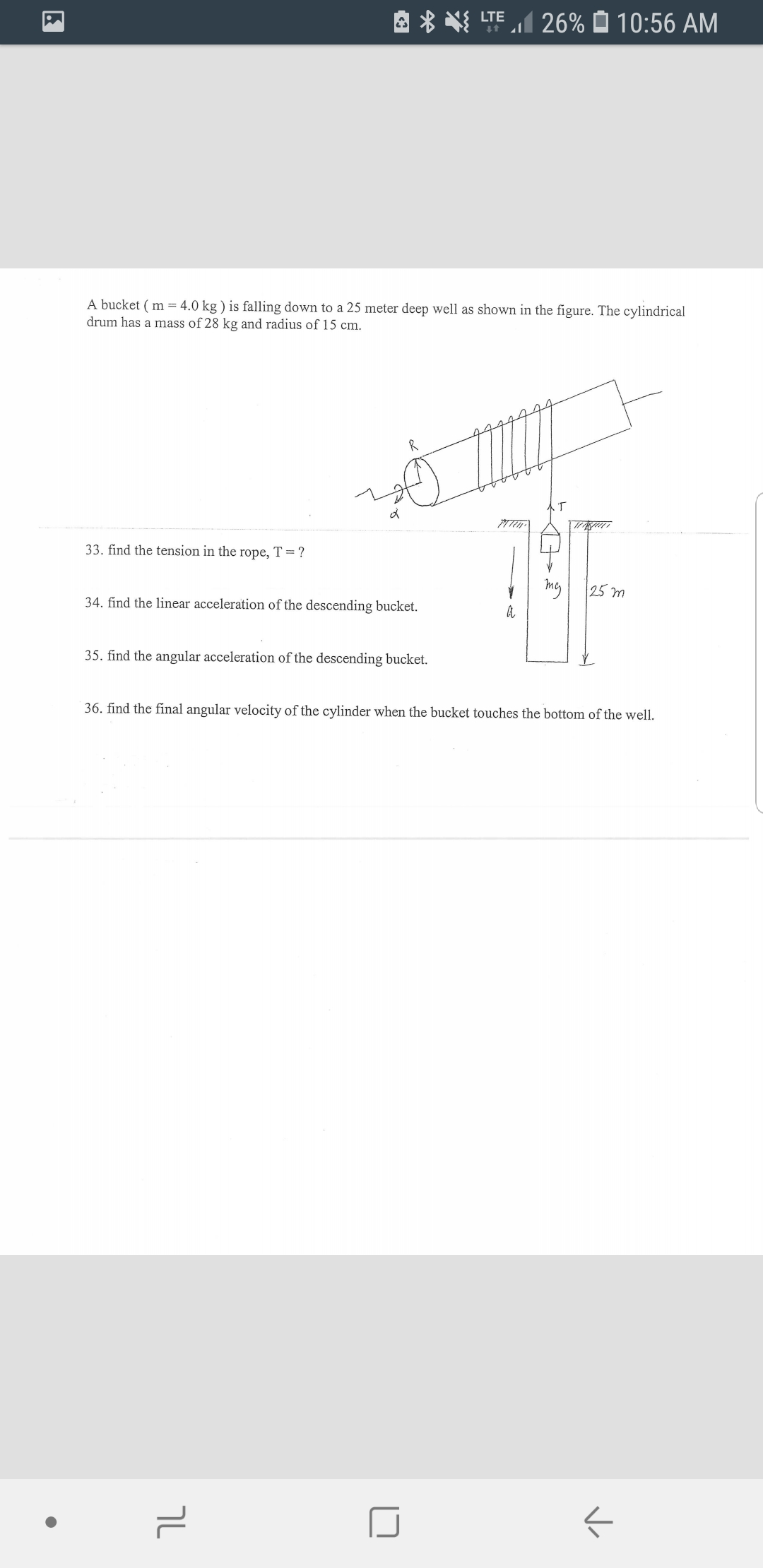# TE26% 10:56 AMA bucket (m 4.0 kg ) is falling down to a 25 meter deep well as shown in the figure. The cylindricaldrum has a mass of 28 kg and radius of 15 cm777733. find the tension in the rope, T = ?25 m34. find the linear acceleration of the descending bucket35. find the angular acceleration of the descending bucket.36. find the final angular velocity of the cylinder when the bucket touches the bottom of the well

Question
20 views

33-36help_outlineImage TranscriptioncloseTE26% 10:56 AM A bucket (m 4.0 kg ) is falling down to a 25 meter deep well as shown in the figure. The cylindrical drum has a mass of 28 kg and radius of 15 cm 7777 33. find the tension in the rope, T = ? 25 m 34. find the linear acceleration of the descending bucket 35. find the angular acceleration of the descending bucket. 36. find the final angular velocity of the cylinder when the bucket touches the bottom of the well fullscreen
check_circle

Step 1

Hello. Since your question has multiple sub-parts, we will solve first three sub-parts for you. If you want remaining sub-parts to be solved, then please resubmit the whole question and specify those sub-parts you want us to solve.

Given information:

Step 2

Here, m and M are the mass of bucket and drum respectively.

d is the depth of the well and R is the radius of the drum.

(33) Consider the FBD’s in the below diagram.

Step 3

Use the FBD of the drum and notice that the tension in the string rotate the drum with angular acceleration α and the MOI of the cylindrical drum is d...

### Want to see the full answer?

See Solution

#### Want to see this answer and more?

Solutions are written by subject experts who are available 24/7. Questions are typically answered within 1 hour.*

See Solution
*Response times may vary by subject and question.
Tagged in

### Rotational Mechanics